# PSEB 4th Class Maths Solutions Chapter 8 Perimeter and Area Ex 8.3

Punjab State Board PSEB 4th Class Maths Book Solutions Chapter 8 Perimeter and Area Ex 8.3 Textbook Exercise Questions and Answers.

## PSEB Solutions for Class 4 Maths Chapter 8 Perimeter and Area Ex 8.3

Question 1.
Colour the area covered by each figure :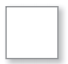Solution: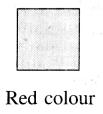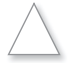Solution: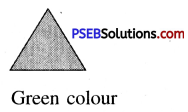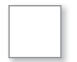Solution: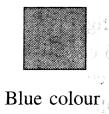Solution: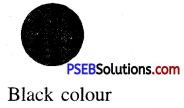Note: You can fill any colour.Question 2.
Find the area of each figure on the basis of number of squares if side of each square is 1 cm and area of each square is 1 square cm.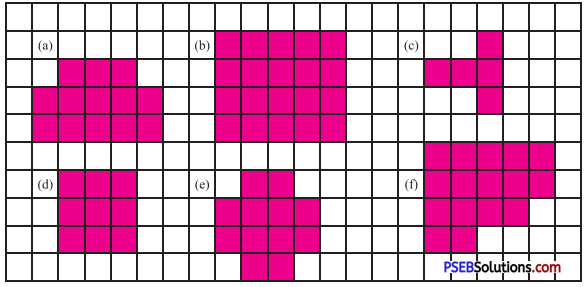Solution:
(a) Number of squares = 13
Area of the figure = 13 × 1 cm2 = 13 cm2

(b) Number of squares = 20
Area of the figure = 20 × 1 cm2 = 20 cm2

(c) Number of squares = 5
Area of the figure = 5 × 1 cm2 = 5 cm2

(d) Number of squares = 9
Area of the figure = 9 × 1 cm2 = 9 cm2

(e) Number of squares = 12
Area of the figure = 12 × 1 cm2 = 12 cm2

(f) Number of squares = 16
Area of the figure = 16 × 1 cm2 = 16 cm2

Question 3.
How many square cm area is covered by each figure ?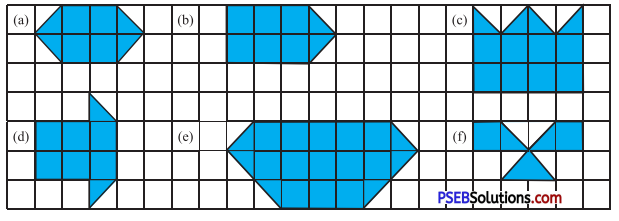Solution:
(a) 6
(b) 7
(c) 10
(d) 7
(e) 13
(f) 4Question 4.
In a notebook with squares draw your favourite figure in which the number of square boxes are:
(a) 20
(b) 27
(c) 15.
Solution:
Try yourself

Question 5.
Look at this picture. Can you divide the figure into four equal parts. How many squares are there in each part?
Solution:
Number of squares = 12
Number of parts into which to be divided = 4
Number of squares in each part = 12 ÷ 4 = 3